# Package Modelica.​Fluid.​UtilitiesUtility models to construct fluid components (should not be used directly)

### Information

Extends from `Modelica.​Icons.​UtilitiesPackage` (Icon for utility packages).

### Package Contents

NameDescription
`checkBoundary`Check whether boundary definition is correct
`cubicHermite`Evaluate a cubic Hermite spline
`cubicHermite_withDerivative`Evaluate a cubic Hermite spline, return value and derivative
`evaluatePoly3_derivativeAtZero`Evaluate polynomial of order 3 that passes the origin with a predefined derivative
`regFun3`Co-monotonic and C1 smooth regularization function
`regPow`Anti-symmetric power approximation with non-zero derivative in the origin
`regRoot`Anti-symmetric square root approximation with finite derivative in the origin
`regRoot2`Anti-symmetric approximation of square root with discontinuous factor so that the first derivative is finite and continuous
`regRoot_der`Derivative of regRoot
`regSquare`Anti-symmetric square approximation with non-zero derivative in the origin
`regSquare2`Anti-symmetric approximation of square with discontinuous factor so that the first derivative is non-zero and is continuous
`regStep`Approximation of a general step, such that the characteristic is continuous and differentiable

## Function Modelica.​Fluid.​Utilities.​checkBoundaryCheck whether boundary definition is correct

### Information

This icon indicates Modelica functions.

Extends from `Modelica.​Icons.​Function` (Icon for functions).

### Inputs

TypeNameDescription
`String``mediumName`
`String``substanceNames[:]`Names of substances
`Boolean``singleState`
`Boolean``define_p`
`Real``X_boundary[:]`
`String``modelName`

## Function Modelica.​Fluid.​Utilities.​regRootAnti-symmetric square root approximation with finite derivative in the origin

### Information

This function approximates sqrt(abs(x))*sgn(x), such that the derivative is finite and smooth in x=0.

FunctionApproximationRange
y = regRoot(x)y ~= sqrt(abs(x))*sgn(x)abs(x) >>delta
y = regRoot(x)y ~= x/sqrt(delta)abs(x) << delta

With the default value of delta=0.01, the difference between sqrt(x) and regRoot(x) is 16% around x=0.01, 0.25% around x=0.1 and 0.0025% around x=1.

Extends from `Modelica.​Icons.​Function` (Icon for functions).

### Inputs

TypeNameDescription
`Real``x`
`Real``delta`Range of significant deviation from sqrt(abs(x))*sgn(x)

### Outputs

TypeNameDescription
`Real``y`

## Function Modelica.​Fluid.​Utilities.​regRoot_derDerivative of regRoot

### Information

Extends from `Modelica.​Icons.​Function` (Icon for functions).

### Inputs

TypeNameDescription
`Real``x`
`Real``delta`Range of significant deviation from sqrt(x)
`Real``dx`Derivative of x

### Outputs

TypeNameDescription
`Real``dy`

## Function Modelica.​Fluid.​Utilities.​regSquareAnti-symmetric square approximation with non-zero derivative in the origin

### Information

This function approximates x^2*sgn(x), such that the derivative is non-zero in x=0.

FunctionApproximationRange
y = regSquare(x)y ~= x^2*sgn(x)abs(x) >>delta
y = regSquare(x)y ~= x*deltaabs(x) << delta

With the default value of delta=0.01, the difference between x^2 and regSquare(x) is 41% around x=0.01, 0.4% around x=0.1 and 0.005% around x=1.

Extends from `Modelica.​Icons.​Function` (Icon for functions).

### Inputs

TypeNameDescription
`Real``x`
`Real``delta`Range of significant deviation from x^2*sgn(x)

### Outputs

TypeNameDescription
`Real``y`

## Function Modelica.​Fluid.​Utilities.​regPowAnti-symmetric power approximation with non-zero derivative in the origin

### Information

This function approximates abs(x)^a*sign(x), such that the derivative is positive, finite and smooth in x=0.

FunctionApproximationRange
y = regPow(x)y ~= abs(x)^a*sgn(x)abs(x) >>delta
y = regPow(x)y ~= x*delta^(a-1)abs(x) << delta

Extends from `Modelica.​Icons.​Function` (Icon for functions).

### Inputs

TypeNameDescription
`Real``x`
`Real``a`
`Real``delta`Range of significant deviation from x^a*sgn(x)

### Outputs

TypeNameDescription
`Real``y`

## Function Modelica.​Fluid.​Utilities.​regRoot2Anti-symmetric approximation of square root with discontinuous factor so that the first derivative is finite and continuous

### Information

Approximates the function

```   y = if x ≥ 0 then sqrt(k1*x) else -sqrt(k2*abs(x)), with k1, k2 ≥ 0
```

in such a way that within the region -x_small ≤ x ≤ x_small, the function is described by two polynomials of third order (one in the region -x_small .. 0 and one within the region 0 .. x_small) such that

• The derivative at x=0 is finite.
• The overall function is continuous with a continuous first derivative everywhere.
• If parameter use_yd0 = false, the two polynomials are constructed such that the second derivatives at x=0 are identical. If use_yd0 = true, the derivative at x=0 is explicitly provided via the additional argument yd0. If necessary, the derivative yd0 is automatically reduced in order that the polynomials are strict monotonically increasing [Fritsch and Carlson, 1980].

Typical screenshots for two different configurations are shown below. The first one with k1=k2=1: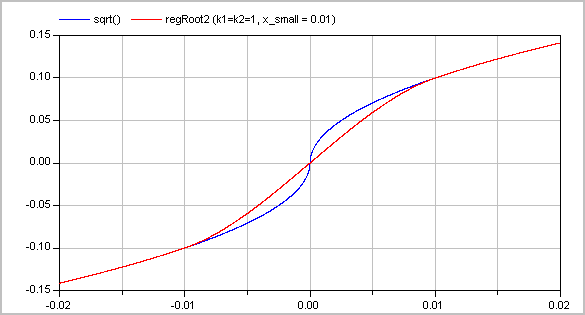and the second one with k1=1 and k2=3: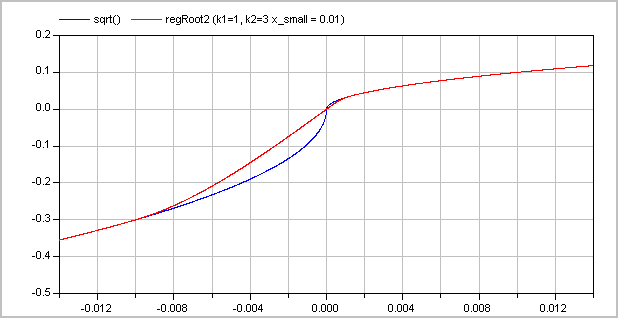The (smooth) derivative of the function with k1=1, k2=3 is shown in the next figure: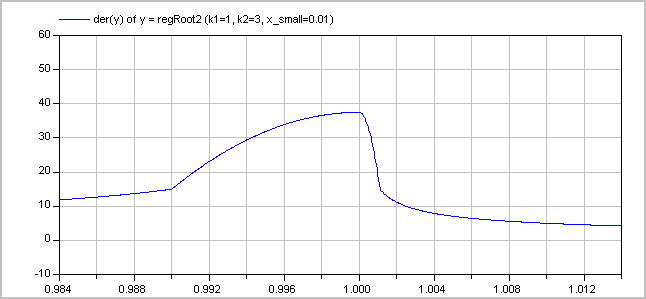Literature

Fritsch F.N. and Carlson R.E. (1980):
Monotone piecewise cubic interpolation. SIAM J. Numerc. Anal., Vol. 17, No. 2, April 1980, pp. 238-246

Extends from `Modelica.​Icons.​Function` (Icon for functions).

### Inputs

TypeNameDescription
`Real``x`abscissa value
`Real``x_small`approximation of function for |x| <= x_small
`Real``k1`y = if x>=0 then sqrt(k1*x) else -sqrt(k2*|x|)
`Real``k2`y = if x>=0 then sqrt(k1*x) else -sqrt(k2*|x|)
`Boolean``use_yd0`= true, if yd0 shall be used
`Real``yd0`Desired derivative at x=0: dy/dx = yd0

### Outputs

TypeNameDescription
`Real``y`ordinate value

## Function Modelica.​Fluid.​Utilities.​regSquare2Anti-symmetric approximation of square with discontinuous factor so that the first derivative is non-zero and is continuous

### Information

Approximates the function

```   y = if x ≥ 0 then k1*x*x else -k2*x*x, with k1, k2 > 0
```

in such a way that within the region -x_small ≤ x ≤ x_small, the function is described by two polynomials of third order (one in the region -x_small .. 0 and one within the region 0 .. x_small) such that

• The derivative at x=0 is non-zero (in order that the inverse of the function does not have an infinite derivative).
• The overall function is continuous with a continuous first derivative everywhere.
• If parameter use_yd0 = false, the two polynomials are constructed such that the second derivatives at x=0 are identical. If use_yd0 = true, the derivative at x=0 is explicitly provided via the additional argument yd0. If necessary, the derivative yd0 is automatically reduced in order that the polynomials are strict monotonically increasing [Fritsch and Carlson, 1980].

A typical screenshot for k1=1, k2=3 is shown in the next figure: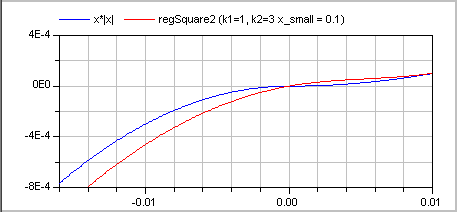The (smooth, non-zero) derivative of the function with k1=1, k2=3 is shown in the next figure: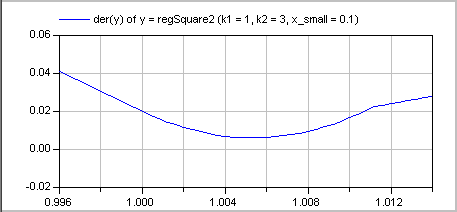Literature

Fritsch F.N. and Carlson R.E. (1980):
Monotone piecewise cubic interpolation. SIAM J. Numerc. Anal., Vol. 17, No. 2, April 1980, pp. 238-246

Extends from `Modelica.​Icons.​Function` (Icon for functions).

### Inputs

TypeNameDescription
`Real``x`abscissa value
`Real``x_small`approximation of function for |x| <= x_small
`Real``k1`y = (if x>=0 then k1 else k2)*x*|x|
`Real``k2`y = (if x>=0 then k1 else k2)*x*|x|
`Boolean``use_yd0`= true, if yd0 shall be used
`Real``yd0`Desired derivative at x=0: dy/dx = yd0

### Outputs

TypeNameDescription
`Real``y`ordinate value

## Function Modelica.​Fluid.​Utilities.​regStepApproximation of a general step, such that the characteristic is continuous and differentiable

### Information

This function is used to approximate the equation

```    y = if x > 0 then y1 else y2;
```

by a smooth characteristic, so that the expression is continuous and differentiable:

```   y = smooth(1, if x >  x_small then y1 else
if x < -x_small then y2 else f(y1, y2));
```

In the region -x_small < x < x_small a 2nd order polynomial is used for a smooth transition from y1 to y2.

Extends from `Modelica.​Icons.​Function` (Icon for functions).

### Inputs

TypeNameDescription
`Real``x`Abscissa value
`Real``y1`Ordinate value for x > 0
`Real``y2`Ordinate value for x < 0
`Real``x_small`Approximation of step for -x_small <= x <= x_small; x_small >= 0 required

### Outputs

TypeNameDescription
`Real``y`Ordinate value to approximate y = if x > 0 then y1 else y2

## Function Modelica.​Fluid.​Utilities.​evaluatePoly3_derivativeAtZeroEvaluate polynomial of order 3 that passes the origin with a predefined derivative

### Information

Extends from `Modelica.​Icons.​Function` (Icon for functions).

### Inputs

TypeNameDescription
`Real``x`Value for which polynomial shall be evaluated
`Real``x1`Abscissa value
`Real``y1`y1=f(x1)
`Real``y1d`First derivative at y1
`Real``y0d`First derivative at f(x=0)

### Outputs

TypeNameDescription
`Real``y`

## Function Modelica.​Fluid.​Utilities.​regFun3Co-monotonic and C1 smooth regularization function

### Information

Approximates a function in a region between `x0` and `x1` such that

• The overall function is continuous with a continuous first derivative everywhere.
• The function is co-monotone with the given data points.

In this region, a continuation is constructed from the given points `(x0, y0)`, `(x1, y1)` and the respective derivatives. For this purpose, a single polynomial of third order or two cubic polynomials with a linear section in between are used [Gasparo and Morandi, 1991]. This algorithm was extended with two additional conditions to avoid saddle points with zero/infinite derivative that lead to integrator step size reduction to zero.

This function was developed for pressure loss correlations properly addressing the static head on top of the established requirements for monotonicity and smoothness. In this case, the present function allows to implement the exact solution in the limit of `x1-x0 -> 0` or `y1-y0 -> 0`.

Typical screenshots for two different configurations are shown below. The first one illustrates five different settings of `xi` and `yid`: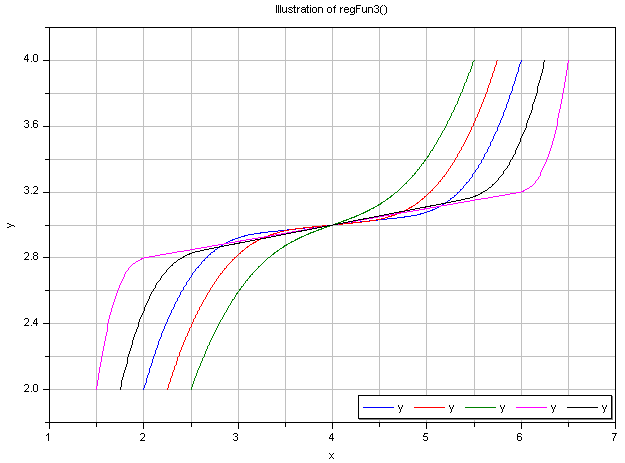The second graph shows the continuous derivative of this regularization function: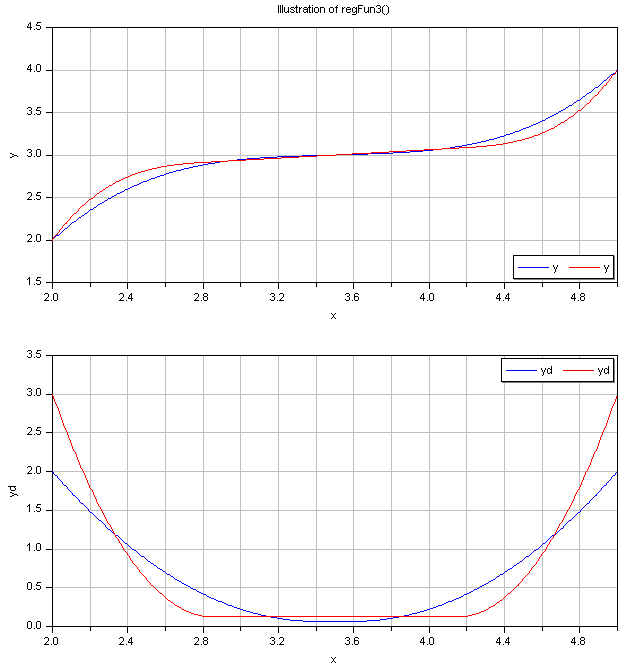Literature

Gasparo M. G. and Morandi R. (1991):
Piecewise cubic monotone interpolation with assigned slopes. Computing, Vol. 46, Issue 4, December 1991, pp. 355 - 365.

Extends from `Modelica.​Icons.​Function` (Icon for functions).

### Inputs

TypeNameDescription
`Real``x`Abscissa value
`Real``x0`Lower abscissa value
`Real``x1`Upper abscissa value
`Real``y0`Ordinate value at lower abscissa value
`Real``y1`Ordinate value at upper abscissa value
`Real``y0d`Derivative at lower abscissa value
`Real``y1d`Derivative at upper abscissa value

### Outputs

TypeNameDescription
`Real``y`Ordinate value
`Real``c`Slope of linear section between two cubic polynomials or dummy linear section slope if single cubic is used

## Function Modelica.​Fluid.​Utilities.​cubicHermiteEvaluate a cubic Hermite spline

### Information

This icon indicates Modelica functions.

Extends from `Modelica.​Icons.​Function` (Icon for functions).

### Inputs

TypeNameDescription
`Real``x`Abscissa value
`Real``x1`Lower abscissa value
`Real``x2`Upper abscissa value
`Real``y1`Lower ordinate value
`Real``y2`Upper ordinate value
`Real``y1d`Lower gradient
`Real``y2d`Upper gradient

### Outputs

TypeNameDescription
`Real``y`Interpolated ordinate value

## Function Modelica.​Fluid.​Utilities.​cubicHermite_withDerivativeEvaluate a cubic Hermite spline, return value and derivative

### Information

This icon indicates Modelica functions.

Extends from `Modelica.​Icons.​Function` (Icon for functions).

### Inputs

TypeNameDescription
`Real``x`Abscissa value
`Real``x1`Lower abscissa value
`Real``x2`Upper abscissa value
`Real``y1`Lower ordinate value
`Real``y2`Upper ordinate value
`Real``y1d`Lower gradient
`Real``y2d`Upper gradient

### Outputs

TypeNameDescription
`Real``y`Interpolated ordinate value
`Real``dy_dx`Derivative dy/dx at abscissa value x

Generated 2018-12-12 12:13:37 EST by MapleSim.